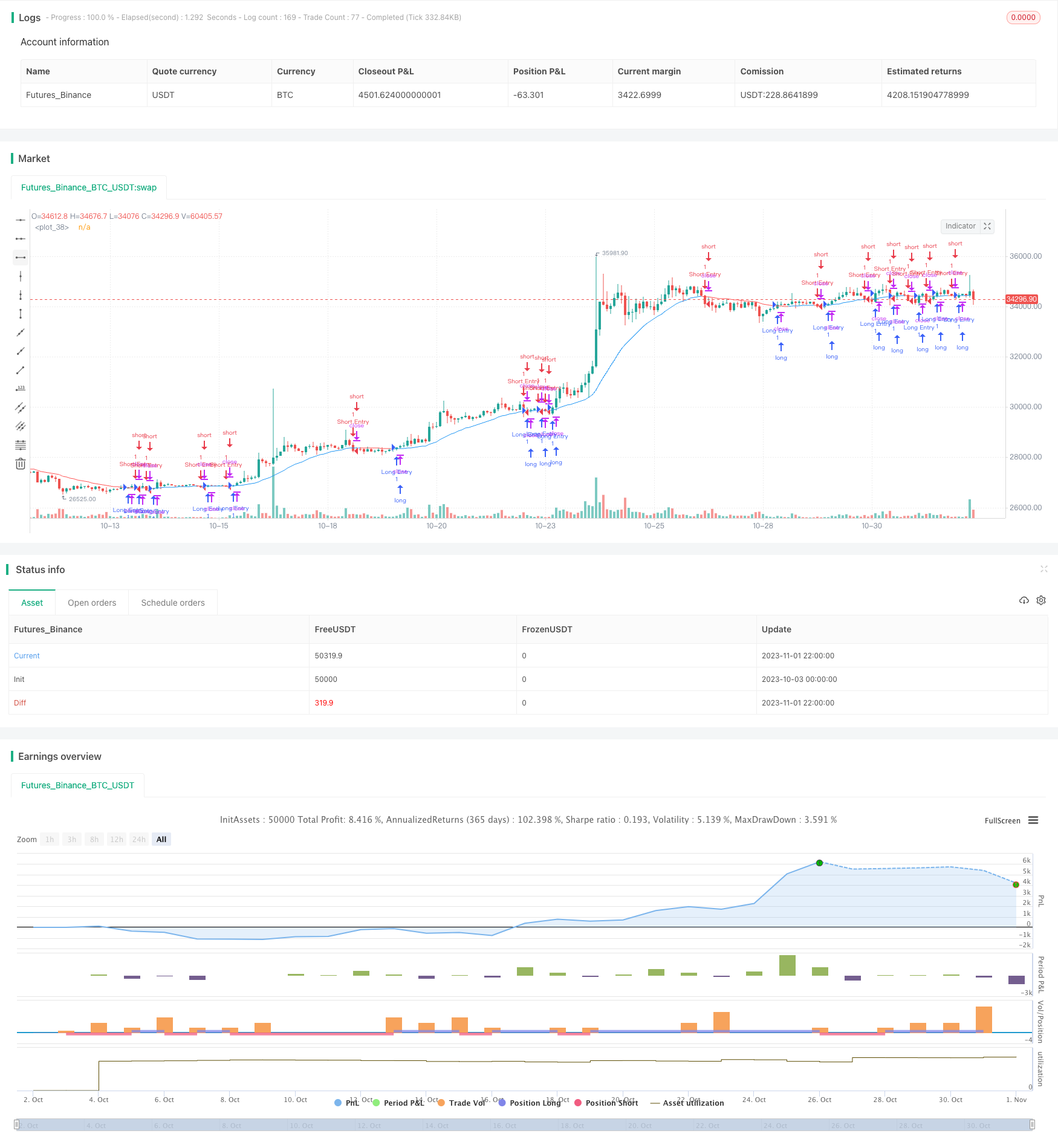# Moving Average Crossover Strategy

Author: ChaoZhang, Date: 2023-11-03 17:23:54
Tags:## Overview

The moving average crossover strategy is a very classic and commonly used technical analysis strategy. The core idea of this strategy is to use the crossover between moving averages of different periods as trading signals. When the short-term moving average crosses above the longer-term moving average from below, a buy signal is generated. When the short-term moving average crosses below the longer-term moving average from above, a sell signal is generated.

## Strategy Logic

This strategy uses input to set the type (SMA, EMA, WMA, RMA) and period of the moving average, as well as the backtesting time range.

Different types of moving averages are calculated in the variant function. The calculated moving average is saved in the ma variable.

When the close price crosses above ma, a buy signal is generated. When the close price crosses below ma, a sell signal is generated.

To set a stop loss, the 14-period average true range atr is calculated. Take the crossover point as a reference, add or subtract 2 times atr as the stop loss range.

The specific entry and exit logic is as follows:

Long entry: close crosses above ma and within backtest time range, stop loss point is entry point close
Long exit: close crosses below ma minus 2 times atr for stop loss exit, or highest price exceeds entry point close plus 2 times atr for take profit exit

Short entry: close crosses below ma and within backtest time range, stop loss point is entry point close
Short exit: close crosses above ma plus 2 times atr for stop loss exit, or lowest price lower than entry point close minus 2 times atr for take profit exit

1. The strategy idea is simple and clear, easy to understand and implement
2. Widely used, suitable for different markets and products
3. Flexible parameter settings, adjustable moving average type and period
4. Use ATR stop loss to help control risks

## Risks of the Strategy

1. Moving average strategies tend to generate frequent trading and stop loss, reducing profit potential
2. In hugely volatile markets, moving averages may generate misleading signals
3. ATR stop loss range could be too wide or too narrow, failing to prevent huge losses

To address the risks, optimizations can be made in the following aspects:

1. Adjust the moving average period, use longer period moving averages
3. Optimize ATR parameters or use other stop loss methods
4. Combine trend indicators to determine overall trend, avoid counter-trend trading

## Optimization Directions

This strategy can be optimized in the following aspects:

1. Add filter conditions such as volume, volatility to avoid irrational breakouts
2. Use adaptive ATR stop loss so the stop loss range changes with market volatility
3. Combine Stoch, RSI and other indicators for multi-factor confirmation to improve signal quality
5. Use time exit to avoid holding losers for too long
6. Optimize moving average period parameters to find best parameter combinations

## Summary

The moving average crossover strategy is a very typical and commonly used technical analysis strategy. The core idea of the strategy is simple and easy to implement, suitable for various markets, and is one of the entry-level quant trading strategies. However, the strategy also has some problems like generating frequent signals and being prone to stop loss. With proper optimizations, the performance can be greatly improved. Overall, the moving average crossover strategy provides a very good framework for strategy development and is the cornerstone of quantitative trading strategy learning.

```/*backtest
start: 2023-10-03 00:00:00
end: 2023-11-02 00:00:00
period: 2h
basePeriod: 15m
exchanges: [{"eid":"Futures_Binance","currency":"BTC_USDT"}]
*/

//@version=4
strategy("MA Cross Strategy", overlay=true,commission_value = 0.1)

type = input(defval = "WMA", title = "MA Type: ", options=["RMA", "SMA", "EMA", "WMA"])

length = input(28)
source = close

// === INPUT BACKTEST RANGE ===
FromDay   = input(defval = 1, title = "From Day", minval = 1, maxval = 31)
FromMonth = input(defval = 1, title = "From Month", minval = 1, maxval = 12)
FromYear  = input(defval = 2000, title = "From Year", minval = 2000)

// === FUNCTION EXAMPLE ===
start     = timestamp(FromYear, FromMonth, FromDay, 00, 00)  // backtest start window
finish    = timestamp(9999, 1, 1, 23, 59)        // backtest finish window
window()  => time >= start and time <= finish ? true : false // create function "within window of time"

variant(type, src, len) =>
v1 = sma(src, len)                                                  // Simple
v2 = ema(src, len)                                                  // Exponential
v5 = wma(src, len)                                                  // Weighted
v7 = rma(src, len)                                                  // Smoothed
type=="EMA"?v2 : type=="WMA"?v5 : type=="RMA"?v7 : v1
ma = variant(type,source, length)

atr = security(syminfo.tickerid, "D", atr(14))

range = valuewhen(cross(close,ma), (atr*2), na)

ep = valuewhen(cross(close,ma), close, na)

plot(ma,color=ma>ma?color.blue:color.red,transp=0,linewidth=1)
plot(ep,color=#2196f3,transp=100,trackprice=true, offset=-9999)
plot(ep+range,color=#2196f3,transp=100,trackprice=true, offset=-9999)
plot(ep-range,color=#2196f3,transp=100,trackprice=true, offset=-9999)

strategy.entry("Long Entry", true, when = crossover(close,ma)  and window() , stop  = ep )
strategy.exit("Long Exit", "Long Entry", stop  = ep-range)
strategy.exit("Long Exit", "Long Entry", when = high > ep+range ,stop = ep )

strategy.entry("Short Entry", false, when = crossunder(close,ma)  and window() , stop  = ep )
strategy.exit("Short Exit", "Short Entry", stop  = ep+range)
strategy.exit("Short Exit", "Short Entry", when = low < ep-range ,stop = ep )

```

More